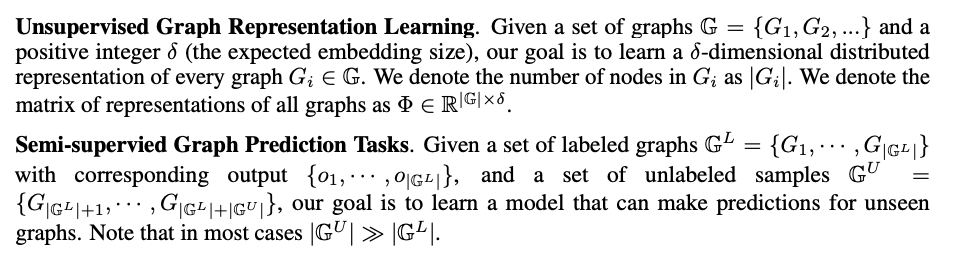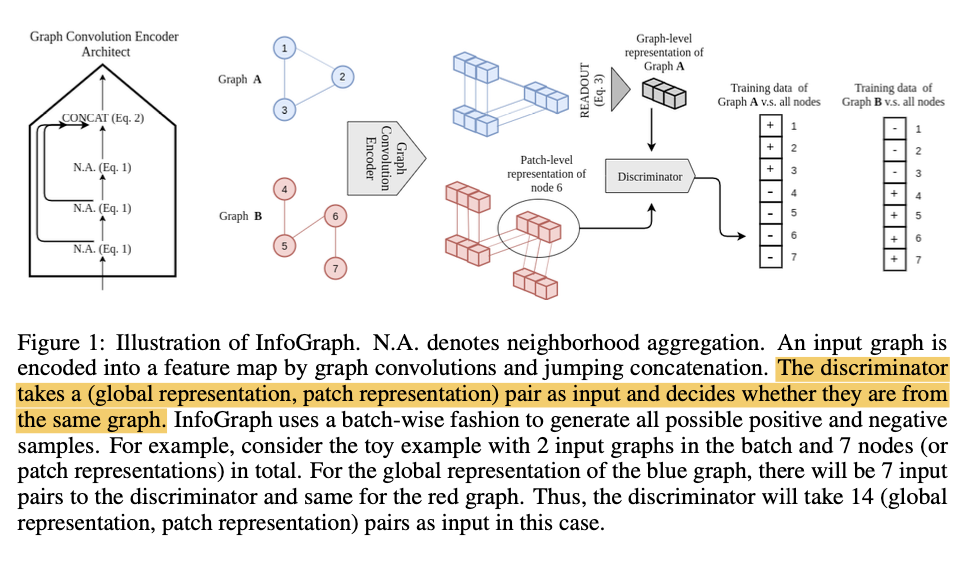N1H111SM's Miniverse

# InfoGraph

2020/05/09 ShareMaterials

# Motivation

Kernel-based methods没有generalization的能力，同时无监督和半监督的setting非常promising。We maximize the mutual information between the graph-level representation and the representations of substructures of different scales (e.g., nodes, edges, triangles).

## Logic of Introduction

• Graph is important, providing diverse structured data.
• Representation learning of the entire graph is a rising field of the community.
• Extant methods are mostly supervised, which is bad since labeling data is costly.
• one way to solve it is through semi-supervised learning.
• or better, by unsupervised learning.
• Introducing representation learning for graphs. They are not to one’s satisfaction since:
• many don’t provide graph embedding explicitly.
• kernels are handcrafted.
• Inspired by the method of maximizing mutual information, we propose InfoGraph.
• Our contribution.
• The graph kernel $K(G1, G2)$ is defined based on the frequency of each sub-structure appearing in $G_1$ and $G_2$ respectively. Namely, $K(G_1, G_2) = \left$, where $f_{G_{s}}$ is the vector containing frequencies of $\{G_s\}$ sub-structures, and $\left< , \right>$ is an inner product in an appropriately normalized vector space.
• An important approach for unsupervised representation learning is to train an encoder to be contrastive between representations that capture statistical dependencies of interest and those that do not.
• Mean Teacher adds a loss term which encourages the distance between the original network’s output and the teacher’s output to be small. The teacher’s predictions are made using an exponential moving average of parameters from previous training steps.

# Model Architecture

## Problem Definition## InfoGraph

In practice, we generate negative samples using all possible combinations of global and local patch representations across all graph instances in a batch.# Experiments

For classification, 6 commonly used datasets.

• MUTAG
• PTC
• REDDIT-BINARY
• REDDIT-MULTI-5K
• IMDB-BINARY
• IMDB-MULTI

For semi-supervised setting, QM9 dataset.